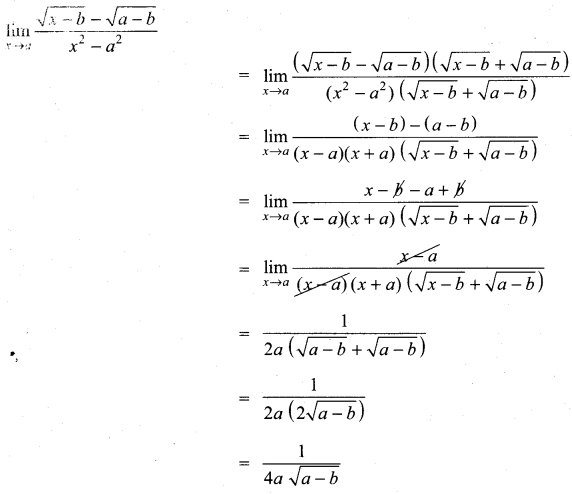Tamilnadu Samacheer Kalvi 11th Maths Solutions Chapter 9 Limits and Continuity Ex 9.2

Evaluate the following limits:
Question 1.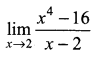Solution: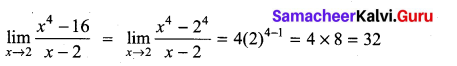Question 2.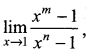m and n are integers.
Solution: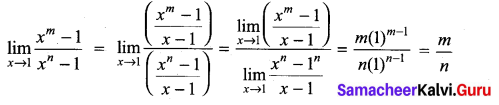Question 3.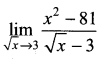Solution: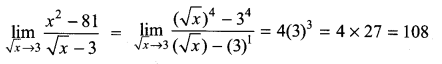Question 4.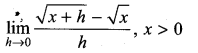Solution: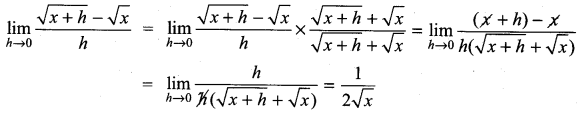Question 5.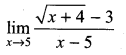Solution: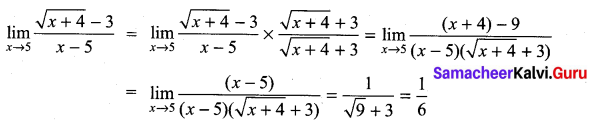Question 6.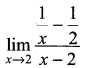Solution: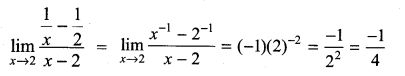Question 7.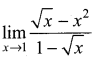Solution: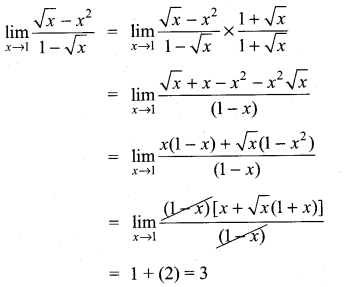Question 8.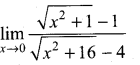Solution: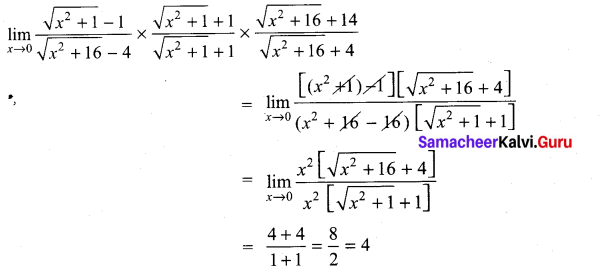Question 9.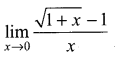Solution: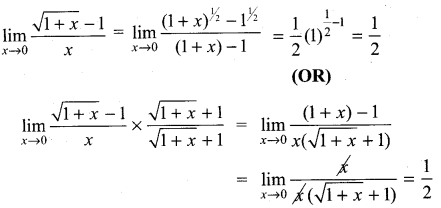Question 10.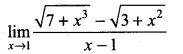Solution: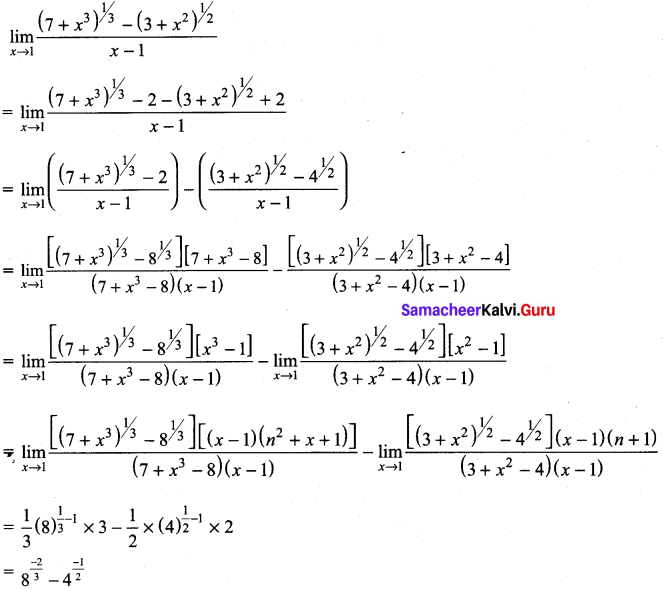= $$\frac{1}{4}-\frac{1}{2}$$
= $$\frac{-1}{4}$$

Question 11.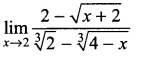Solution: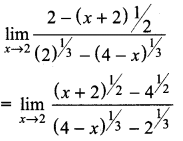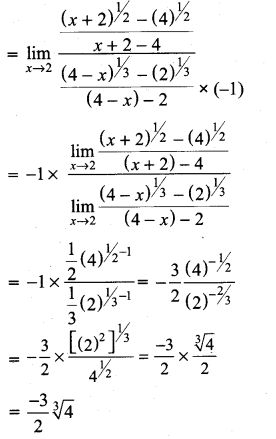Question 12.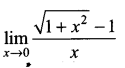Solution: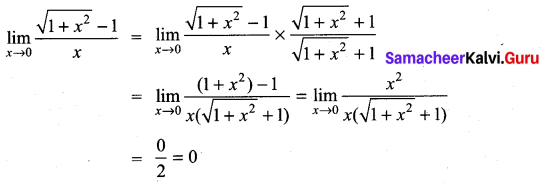Question 13.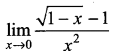Solution: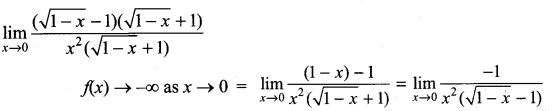∴ Limit does not exist

Question 14.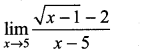Solution: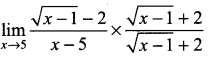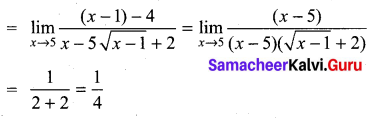Question 15.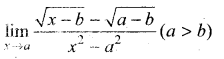Solution: